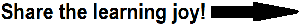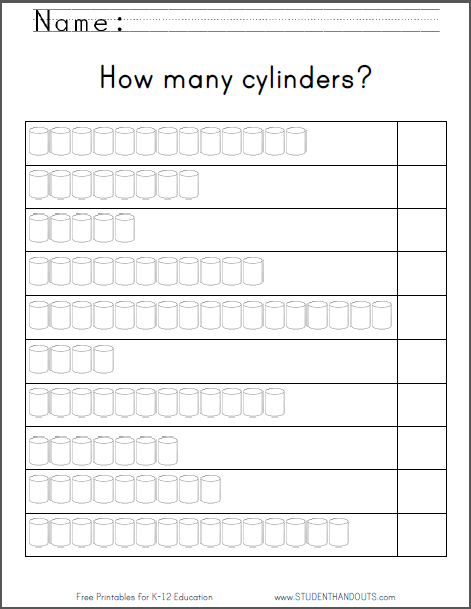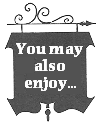Cylinders Counting Worksheet for Kindergarten | Student HandoutsCylinders Counting WorksheetStudents count the number of cylinders, then write the correct number in the corresponding box. Numbers range up to seventeen. Click here to print this worksheet.

 CCSS.MATH.CONTENT.K.CC.A.3 - Write numbers from 0 to 20. Represent a number of objects with a written numeral 0-20 (with 0 representing a count of no objects).
 CCSS.MATH.CONTENT.K.CC.B.5 - Count to answer "how many?" questions about as many as 20 things arranged in a line, a rectangular array, or a circle, or as many as 10 things in a scattered configuration; given a number from 1-20, count out that many objects.